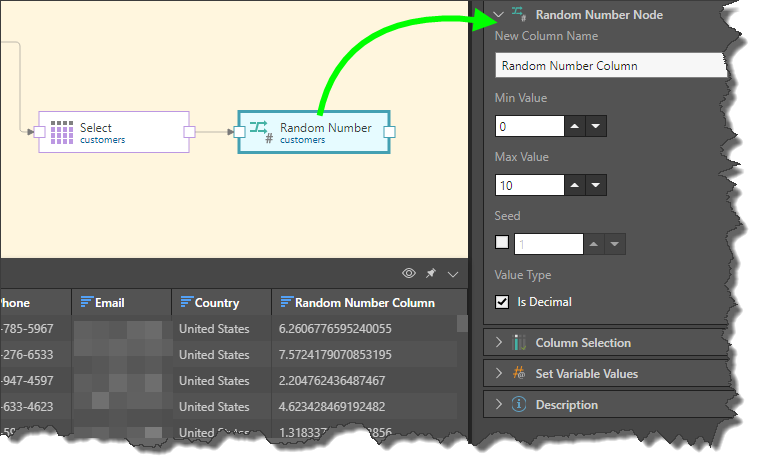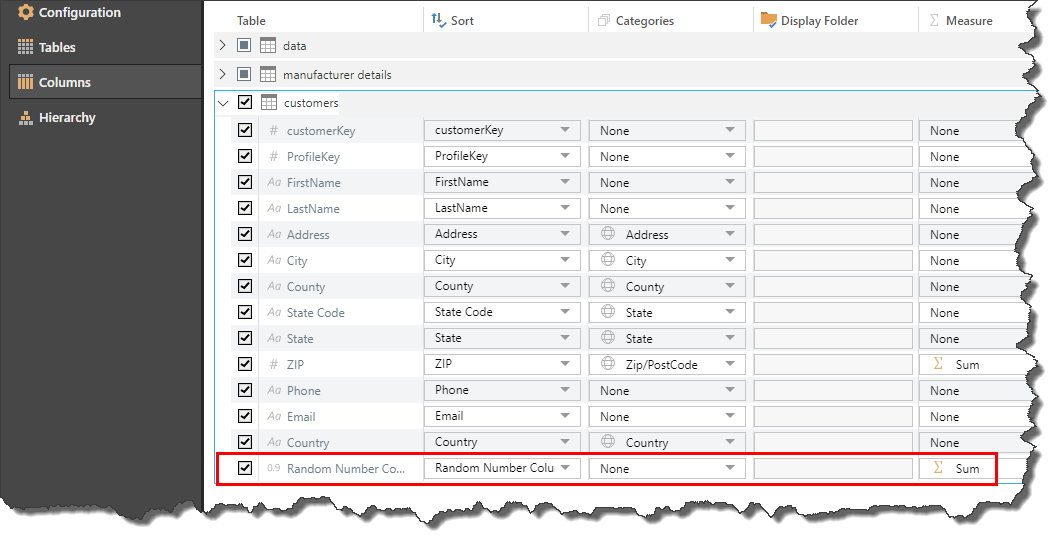# Random Number

Add a new column to the given table containing random numbers. This differs from the Add Sequence node, as the numbers are random, not sequential. Random numbers are used for a variety of purposes, including random sampling, testing of formulas in a simulation, randomly sorting data, and more.

## How to Configure a Random Number Node

Connect the Random Number node to the Select node representing the relevant table. Go to the Properties panel to configure the node:

• New Column Name: enter the name of the new random number column.
• Min Value: set the minimum random number value.
• Max Value: set the maximum random number value.
• Seed: select this option if you want the random numbers to remain static when the preview is refreshed or the ETL re-run. See below for details.
• Value Type: select Is Decimal if you want the random numbers to contain a decimal point.By default, the new Random Number column will be assigned as a measure. If you want it to be used as a column, go to Tables or Columns and make the column visible (by selecting its check box) and assign it to the measure type 'None'.### Seed

By default, when the preview is refreshed or the ETL is run again, the numbers generated for each row by the Random Number node will be refreshed. This means that each time the ETL is run the number for each row will be changed, and this will be reflected in any existing data discoveries containing the random number column.

If you want the random number column to remain static whenever the ETL is run, enable Seed from the node's Properties panel. When the seed is enabled, it must be assigned a numeric value, which determines the starting point of the random numbers. The default seed value is 1, but you may enter any value here.

As long as the seed value is not disabled or changed, the random numbers will remain static whenever the preview is refreshed or the ETL is run. If additional rows are added to the table, the existing rows will retain their original random number, and a new random number will be assigned to each new row.

### Example

Here the Random Number column was assigned a max value or 1000 and a seed value of 5: